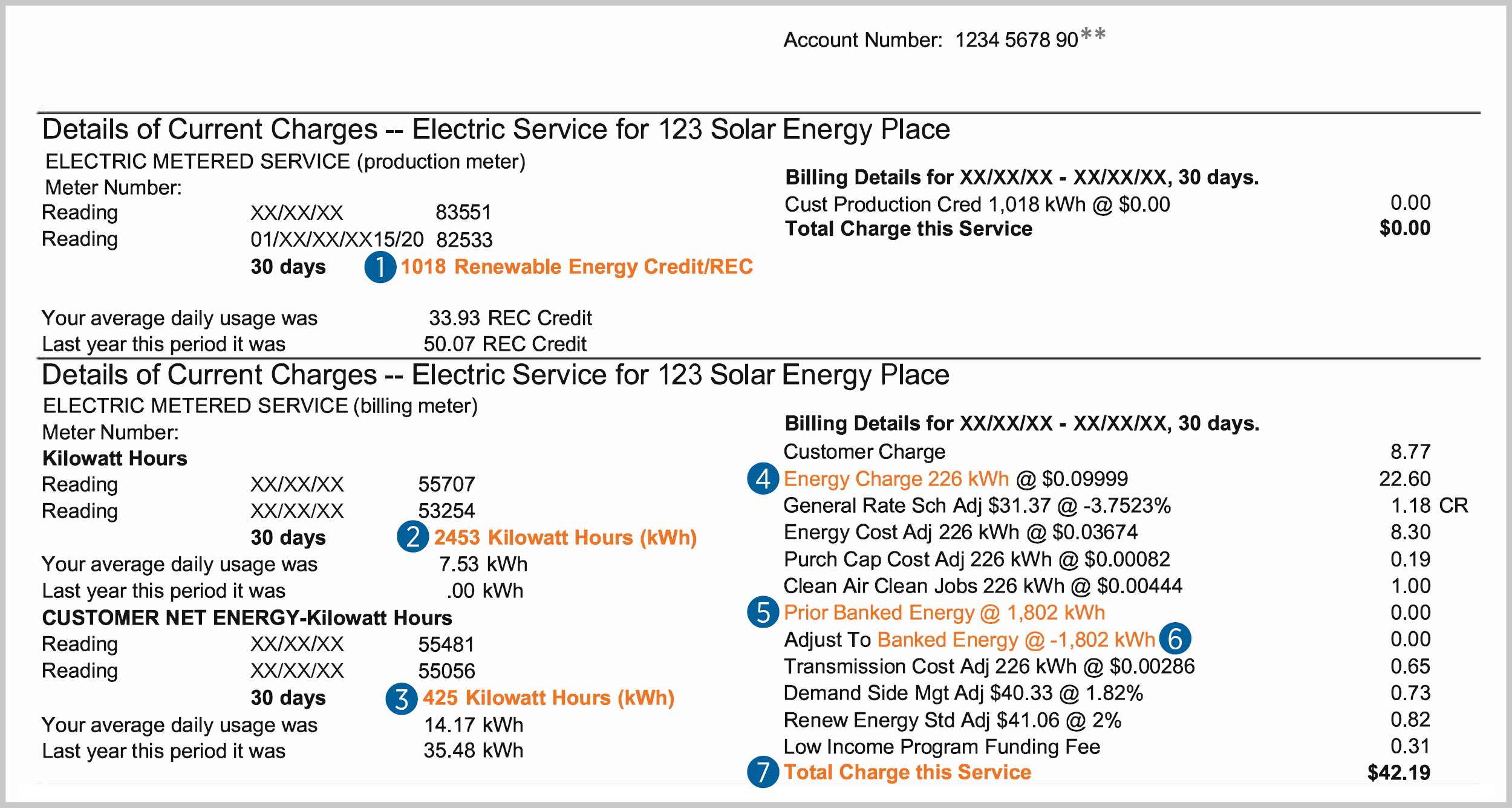#When your solar system produces energy, you begin using this energy right away, in real time. You may not be using all of it, and some of it goes back to the Black Hills Energy grid. This amount of energy will offset your total energy bill this month and potentially in the future depending on the production of your system.

## Important components of your solar bill1. Renewable Energy Credit*: 1018 kWh - this is the total energy production of your solar system during the billing period. This is the sum of the energy you use in real time plus the amount of energy that goes back to the Black Hills Energy grid.

2. Kilowatt Hours Reading: 2453 kWh - this is how much energy you needed from Black Hills Energy during the billing period. This is the energy you needed primarily during the night or when your solar system was not producting energy.

3. Customer Net Energy: 425 kWh - this is the amount of excess energy your solar system produced - more than you needed during the day.

4. Energy Charge: 226 kWh- this is what you owe on your current bill. To calculate: Kilowatt Hours Reading (2453 kWh) minus Customer Net Energy (425 kWh) minus Prior Banked Energy (1802 kWh) = 226 kWh.

5. Prior Banked Energy: 1802 kWh - this is the amount of energy from your solar system that was "banked," or carried over from the previous month. This varies greatly from month to month and can be zero.

6. Banked Energy: 1802 kWh - this is the amount of banked energy that's applied to your bill. This amount is subtracted from your "bank."

7. Total Charge this Service: \$42.19 - this is the total amount due on your bill.

*In some cases, customers may not have a Renewable Energy Credit on their bill. This could be due to a third-party owning the solar system or as a result of not having a production meter.

**This example shows a portion of the total bill.

### Energy you produce and use from your solar system

A. Renewable Energy Credit - this is the total energy production of your solar system during the billing period. In this example, that amount is 1018 kWh. This is the sum of the energy you use in real time plus the amount of excess energy that goes back ot the Black Hills Energy grid.

B. Customer Net Energy - this is the amount of excess energy your solar system produced - more than you needed during the day. In this example, your solar system produced 425 kWh of excess energy. This energy is sent to the Black Hills Energy grid and is used to off-set the amount of energy you need from the grid.

### Energy you use from Black Hills Energy's grid

C. Kolowatt Hours Reading (kWh) - 2453 kWh - this represents how much energy you needed from Black Hills Energy during the billing period. This is the energy you needed primarily during the night or when your solar system was not producing energy.

### Sum it up

D. Energy used in real-time - 593 kWh - this is the energy produced from your solar system, used in real-time to serve your day-time energy needs.

1018 kWh - 425 kWh = 593 kWh

E. Total energy used in your home during the billing period - 2621 kWh - this is calculated by the sum of the energy your solar system produced and used during the day (593 kWh), plus the Calculation: 2453 kWh - 425 kWh = 2028 kWh.

Total net energy used: 593 kWh (D) + 2453 kWh (C) - 425 kWh (B) = 2621 kWh (E)